# Count the number of cells in a range in Microsoft Excel 2010

There could be times when you have a huge range of cells in a column or multiple columns and you need to count the number of entries in all the cells put together. You can do this using one formula itself instead of using many count formulae together.

Count Cells with Numbers (COUNT FUNCTION)

The COUNT function counts the number of cells that contain numbers & ignores any text. The COUNT function is used to get the number of entries in a number field that is in a range or array of numbers.

Syntax

COUNT(value1, [value2], ...)

The COUNT function syntax has the following arguments:

value1 Required. The first item, cell reference, or range within which you want to count numbers.

value2, ... this is optional & we can have up to 255 additional items or cell references to count numbers.

The arguments (e.g. value1) can be cell references, or values typed into the COUNT formula.

• In cell A7 enter formula as =COUNT(A1:A5)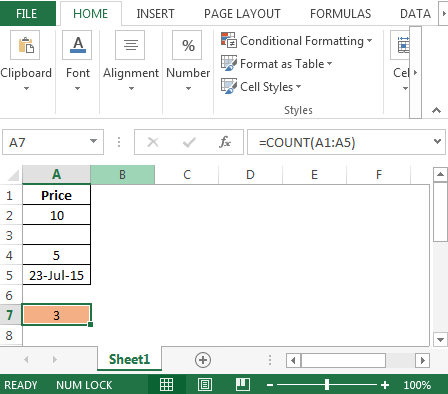• The result is 3 because there are 3 numbers in the above range
• Cell A1 will not be consider because it contains text
• Cell A5 is included because dates are stored as numbers

Count Cells with Data (COUNTA FUNCTION)

The COUNTA function will count cells that are not empty.

Its syntax is: =COUNTA(value1, value2,...)

The arguments (e.g. value1) can be cell references, or values typed into the formula.

• In cell A7 enter formula as =COUNTA(A1:A5)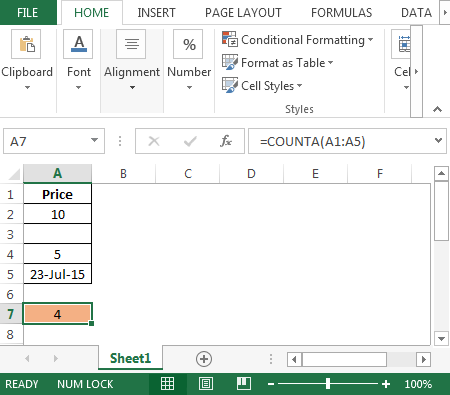• The result is 4 because there are 4 cells having text or value

Count Blank Cells (COUNTBLANK FUNCTION)

The COUNTBLANK function will count cells that are empty.

Its syntax is: =COUNTBLANK(range)

• In cell A7 enter formula as =COUNTBLANK(A1:A5)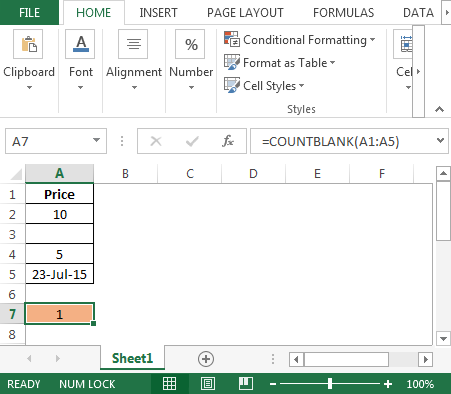• The result will be 1 as there is only one cell is empty i.e. cell A3

Count the number for Rows

Lets consider we have data in the range A2:A10.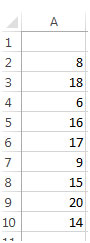We need to count the number of values in this range. In cell C2, put this formula –

=COUNT(A2:A10)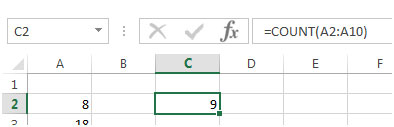We get the result as 9.

Count the number for Columns

Here, the data was present in a column. Similarly, if we have data in one row, like this –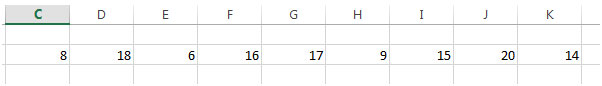We can enter the formula in C4 as –

=COUNT(C2:K2)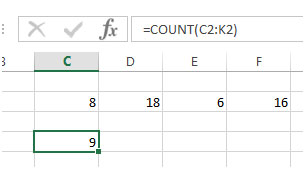The result we get is 9 again.

Count the number for Rows and Columns

Now, if we have a bigger range of data, covering multiple rows and columns, for example, consider that we have data in the range A1:E20 –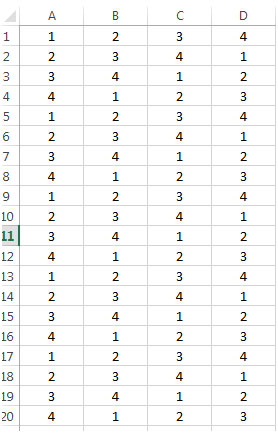We need to count the values in this range. All we need to do is, put this formula in a blank cell in the sheet –

=COUNT(A1:D20)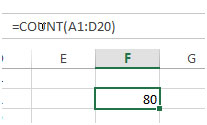The result we get is 80 which is the count of all the cells in A1:D20.

Now if we remove the values from some cells, this number will automatically get updated and show us the current value.